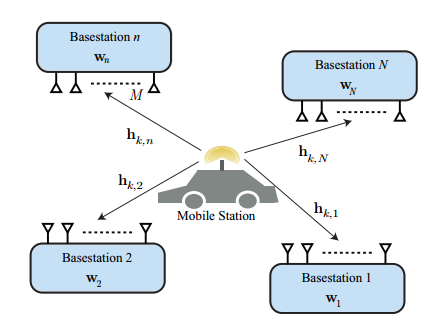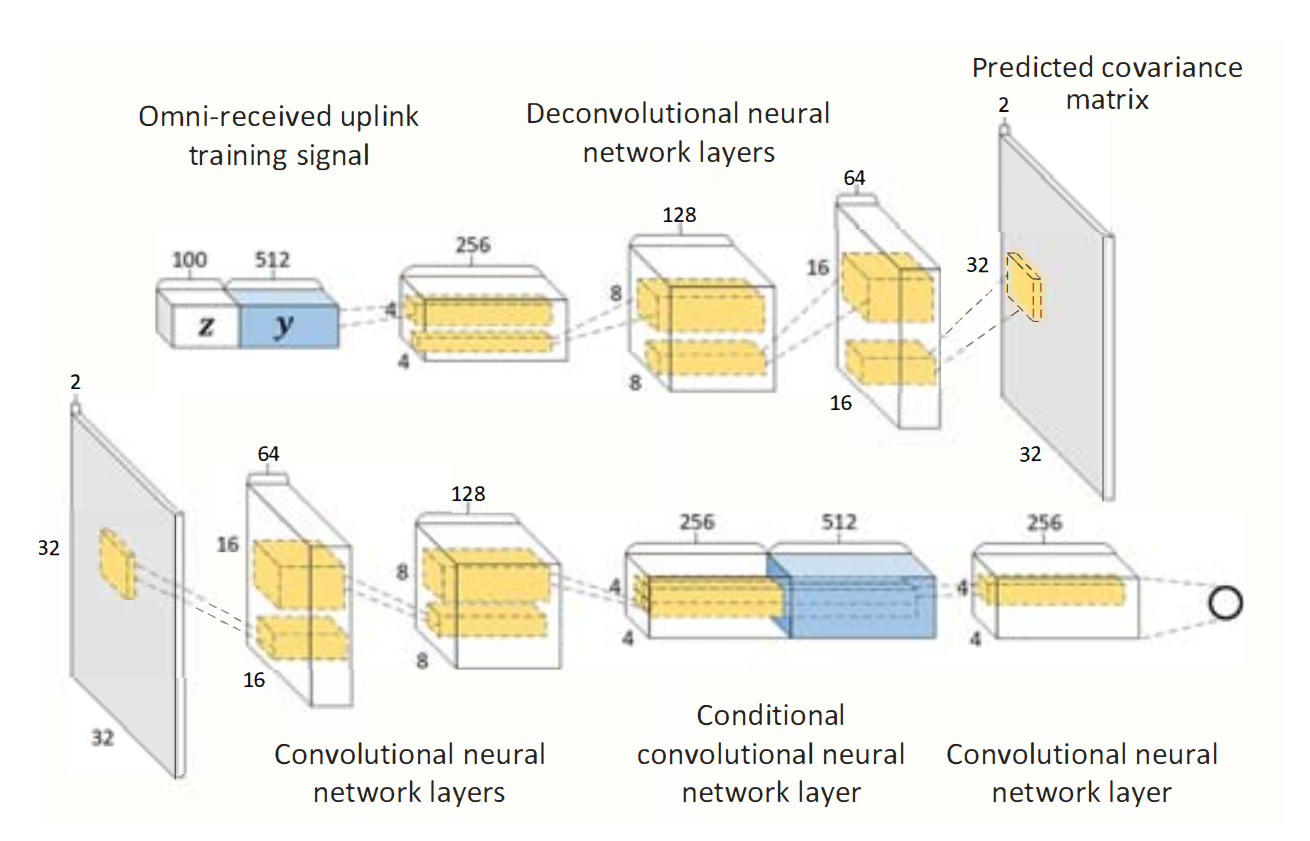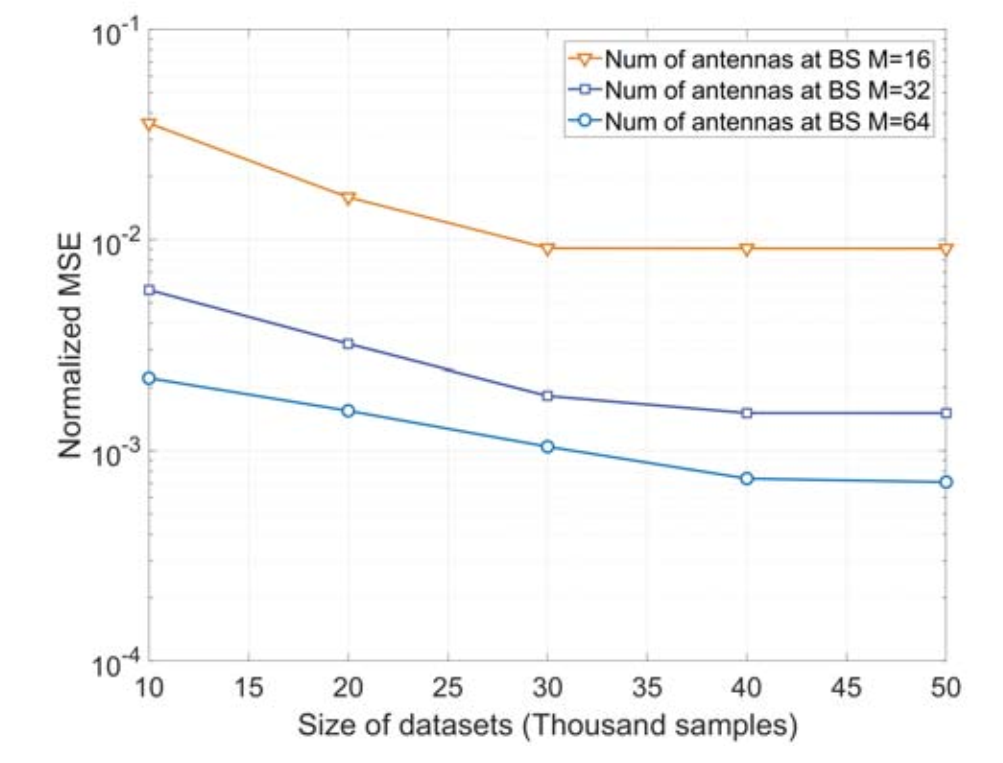# 系统模型## 信道模型

h d , n = M ρ ∑ ℓ = 1 L g n , ℓ p ( d T s − τ n , ℓ ) a ( θ n , ℓ ) \boldsymbol{h}_{d, n}=\sqrt{\frac{M}{\rho}} \sum_{\ell=1}^{L} g_{n, \ell} p\left(d T_{s}-\tau_{n, \ell}\right) \mathbf{a}\left(\theta_{n, \ell}\right)

h k , n = ∑ d = 0 D − 1 h d , n e − j 2 π k K d ， \mathbf{h}_{k, n}=\sum_{d=0}^{D-1} \boldsymbol{h}_{d, n} e^{-j \frac{2 \pi k}{K} d}，

y k , n = w n T h k , n s k + v k y_{k, n}=\mathbf{w}_{n}^{T} \mathbf{h}_{k, n} s_{k}+v_{k}

## 网络相关

• 通过所有基站上收到的pilot， 估计信道。因此，输入向量可写为 y = [ y 1 , 1 , ⋯ &ThinSpace; , y K , 1 , y 1 , 2 , ⋯ &ThinSpace; , y K , 2 , ⋯ &ThinSpace; , y K , N ] T \mathbf{y}=\left[y_{1,1}, \cdots, y_{K, 1}, y_{1,2}, \cdots, y_{K, 2}, \cdots, y_{K, N}\right]^{T}
也就是所有基站所有子载波上的一个pilot的结合。
如同在Deep Learning Coordinated Beamforming for Highly-Mobile Millimeter Wave Systems提出的一样，这篇文章沿用了 **Omni-antenna patterns：

In , the authors showed that when the uplink training pilots are received simultaneously by multiple distributed basestations using omni or quasi-omni antenna patterns, these omni-received signals draw a rich multipath signature for the user location and its interaction with the surrounding environment.

w n = [ 1 , 0 , … , 0 ] , ∀ n \mathbf{w}_{n}=[1,0, \dots, 0], \forall n

• 不需要设计接受波束成形向量了，很方便。

## 稀疏变换

R h n = E [ h k , n h k , n H ] \mathbf{R}_{\mathbf{h}_{n}}=\mathbb{E}\left[\mathbf{h}_{k, n} \mathbf{h}_{k, n}^{H}\right]

h d , n = U B S g d , n \boldsymbol{h}_{d, n}=\mathbf{U}_{\mathrm{BS}} \boldsymbol{g}_{d, n}
U B S \mathbf{U}_{\mathrm{BS}} 是DFT矩阵。 由于空间中路径数十分有限，因此 g d , n \boldsymbol{g}_{d, n} 中绝大部分元素都为0。（这一部分看不懂的同学可以查阅下作者给出的引文《An Overview of Signal Processing Techniques for Millimeter Wave MIMO Systems》）。

R h n = U B S R g n U B S H \mathbf{R}_{\mathbf{h}_{n}}=\mathbf{U}_{\mathrm{BS}} \mathbf{R}_{\mathrm{g}_{n}} \mathbf{U}_{\mathrm{BS}}^{H}

it is much easier to learn the mapping from the omni-received uplink signature y to the virtual channel covariance matrices as compared to the original channel covariance matrix.

# 网络模型• 通过导频序列，生成 R g n \mathbf{R}_{\mathrm{g}_{n}} 。 可以理解为导频通过神经网络，输出了信道预测值。
本文和其他许多基于深度学习的信道估计文章不同， 其他文章往往以准确信道信息为标签，并计算网络输出值与真实值的MSE作为loss，不断训练网络缩小loss， 而本文则是通过GAN的方式来完成训练：
min ⁡ G max ⁡ D L ( G , D ) = E R ε n [ log ⁡ D ( R g n ∣ y ) ] + E z [ log ⁡ ( 1 − D ( G ( z ∣ y ) ) ) ] \begin{aligned} \min _{G} \max _{D} L(G, D) =\mathbb{E}_{\mathbf{R}_{\mathbf{\varepsilon}_{n}}}\left[\log D\left(\mathbf{R}_{\mathbf{g}_{n}} | \mathbf{y}\right)\right] +\mathbb{E}_{\mathbf{z}}[\log (1-D(G(\mathbf{z} | \mathbf{y})))] \end{aligned}
这是GAN的经典公式： 先固定生成器G， 训练最优的判别器D来区分真实样本和生成样本。 在这里，分别代表了 真实信道信息和估计（生成）的信道信息。 然后，固定判别器D， 训练生成器，使得估计的信道信息尽可能贴近于真实信道信息。 来回迭代，直至收敛。
GAN相关的推导可以参考这里 G ( z ∣ y ) ) G(\mathbf{z} | \mathbf{y})) 表示在接收的导频序列为 y \mathbf{y} 的情况下，通过噪声 z \mathbf{z} 生成（估计）的 R g n \mathbf{R}_{\mathbf{g}_{n}} , 也可以表示为 R ^ g n = G ( z , y ) \hat{\mathbf{R}}_{\mathbf{g}_{n}}=G(\mathbf{z}, \mathbf{y})

# 仿真结果

N M S E = ∥ R ^ g n − R g n ∥ F 2 ∥ R g n ∥ F 2 \mathrm{NMSE}=\frac{\left\|\hat{\mathbf{R}}_{\mathrm{g}_{\mathrm{n}}}-\mathbf{R}_{\mathrm{g}_{\mathrm{n}}}\right\|_{\mathrm{F}}^{2}}{\left\|\mathbf{R}_{\mathrm{g}_{\mathrm{n}}}\right\|_{\mathrm{F}}^{2}}03-11359212-09465
01-01228
04-123649
12-2068
07-113125
07-305235
02-081万+
09-221万+
05-013万+
07-204076
09-191993
09-172215
11-21155
08-311万+